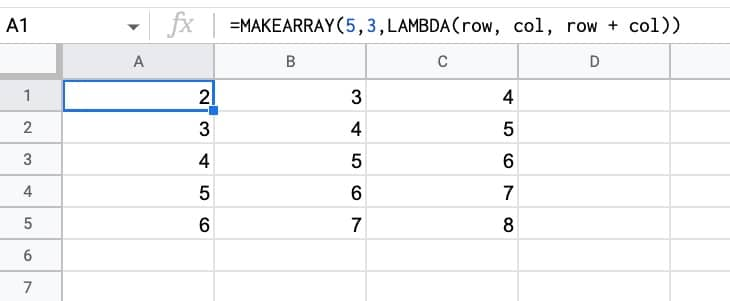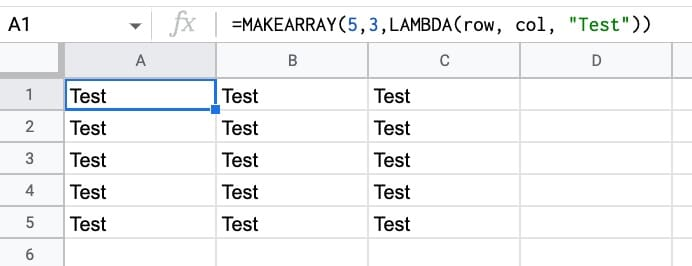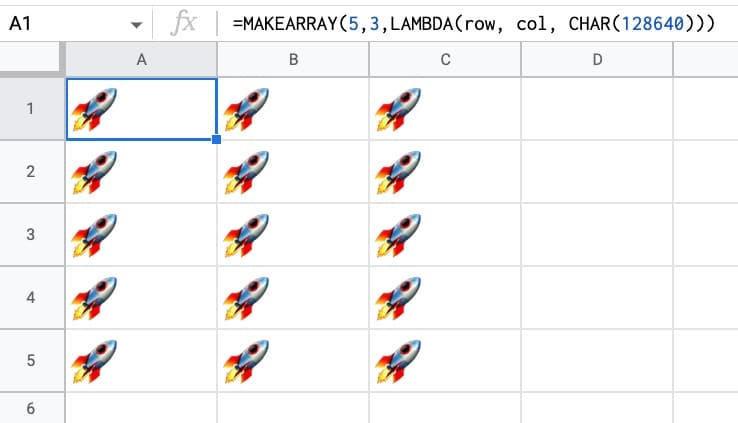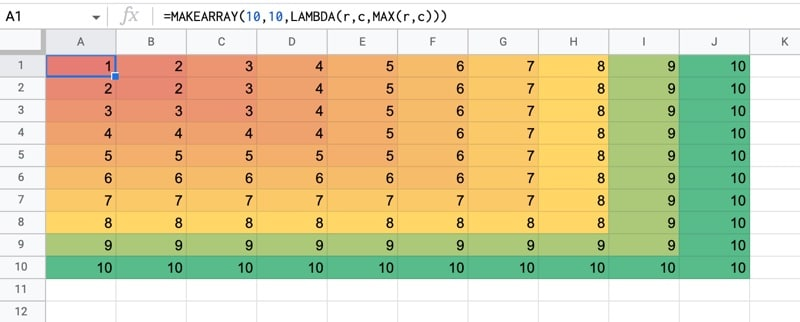# MAKEARRAY Function in Google Sheets – LAMBDA Helper Function

The MAKEARRAY function in Google Sheets generates an array of a specified size, with each value calculated by a custom lambda function.

The Lamba function has access to the row and column indices for each value.

Let’s see a simple example:

`=MAKEARRAY(5,3,LAMBDA(row, col, row + col))`

This generates an array with 5 rows and 3 columns.

The value of each element in the row is the sum of the row position and the column position, within the array.

So the first value is 2 (row 1 + column 1) and the final value is 8 (row 5 + column 3). The indices are in relation to the position within the array, not the position within the Google Sheet.🔗 Get this example and others in the template at the bottom of this article.

## MAKEARRAY Function Syntax

`=MAKEARRAY(rows, columns, lambda)`

It takes three arguments:

`rows`

The number of rows in the array generated by the MAKEARRAY function.

`columns`

The number of columns in the array generated by the MAKEARRAY function.

`lambda`

This argument is the custom LAMBDA function that will be applied to each value in the array. The LAMBDA function must have 2 arguments, representing the row and column index of each value, and 1 function expression.

### MAKEARRAY Function Notes

The SEQUENCE function also generates numeric arrays and is easier to use.

However, MAKEARRAY can create more complex array structures.

## MAKEARRAY Function With Static Output Value

We can set the lamba expression to return a constant value, rather than compute something with the row and column index values.

For example, our MAKEARRAY could generate an array of fixed values:

`=MAKEARRAY(5,3,LAMBDA(row, col, 99))`

This generates an array, 5 rows by 3 columns, consisting of the value 99 in each position. The lambda ignores the row and column indices and returns 99 for each iteration.

Similarly, this next MAKEARRAY formula generates a 5 by 3 array of strings “Test”:And of course, you can use the CHAR function to generate a funky symbol to repeat in your array:

`=MAKEARRAY(5,3,LAMBDA(row, col, CHAR(128640)))`

Which generates an array of rockets!## MAKEARRAY Formula With MAX Function

We can of course create more complex lamba expressions, using the row and column indices.

For example, this formula will create a 10 by 10 array with the max value of the row and column indices for each position in the array.

`=MAKEARRAY(10,10,LAMBDA(r,c,MAX(r,c)))`

You can see the lambda function which calculates the maximum of the r or c values (the row or column values).

Here’s the output, with a heatmap added to highlight the different values:## MAKEARRAY Function Template

Feel free to make a copy: File > Make a copy…

If you can’t access the template, it might be because of your organization’s Google Workspace settings.

In this case, right-click the link to open it in an Incognito window to view it.

1.James Murray says: